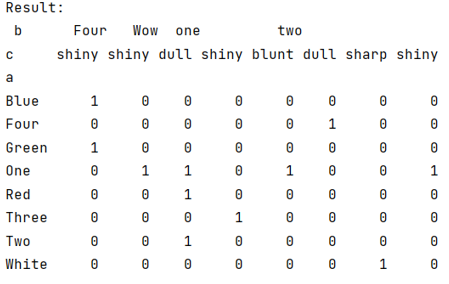# Pandas crosstab() function with example

Learn about the Pandas crosstab() function with example. By Pranit Sharma Last updated : September 24, 2023

Pandas is a special tool that allows us to perform complex manipulations of data effectively and efficiently. Inside pandas, we mostly deal with a dataset in the form of DataFrame. DataFrames are 2-dimensional data structures in pandas. DataFrames consist of rows, columns, and data.

Here, we will learn about the Pandas.crosstab() method, its usage, syntax, and examples.

## Pandas.crosstab() Method

The crosstab() method is just like the pivot function and somewhere it used the concept of the pivot table.

## Syntax

```pandas.crosstab(
index,
columns,
values=None,
rownames=None,
colnames=None,
aggfunc=None,
margins=False,
margins_name='All',
dropna=True,
normalize=False
)
```

## Parameter(s)

• index: an array, series or values to be grouped.
• columns: values of columns to be grouped.
• values: optional parameter, an array of some elements to be operated on aggregate function.
• aggfunc: an optional function, it requires a 'value' parameter on which this function will work.

Let us understand with the help of an example,

## Example of pandas.crosstab() function

```# Importing pandas package
import pandas as pd

# Importing numpy package
import numpy as np

# Creating numpy arrays
a = np.array(["One", "Two", "Three", "Four",
"Red", "Blue", "Green", "White",
"One", "One", "One"],
dtype=object)

b = np.array(["one", "one", "one", "two",
"one", "Four", "Four", "two",
"two", "two", "Wow"],
dtype=object)

c = np.array(["dull", "dull", "shiny",
"dull", "dull", "shiny",
"shiny", "sharp", "shiny",
"blunt", "shiny"],
dtype=object)

# Performing the cross tab
result = pd.crosstab(a, [b, c], rownames=['a'], colnames=['b', 'c'])

# Display result
print("Result:\n",result)
```

### Output

The output of the above program is: